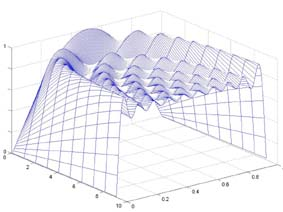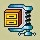MATHEMATICAL METHODS IN ENGINEERING Summer Term 2012 / 2013 Room A335: Tuesday 9:00-12:30 Assessment: 2 / 0 / 2   z, Zk Lectures: Prof. Ing. Aleš Procházka, CSc                  Laboratories: Ing. Mohammadreza Yadollahi

Week Lectures Laboratories   Texts   Files    Projects
1 Introduction: Software support of mathematical methods, numerical, symbolical and simulation tools. MATLAB environment: basic operations, commands, matrix operations, programming methods, fundamental algorithms MATLAB: Interactive and programming methods, selected functions and commands, editor, programming2 MATLAB environment: Two-dimensional and three-dimensional graphics, visualization, object hierarchy, color selection, data import and export, GUI MATLAB: Visualization of functions, definition of color sets, data import, GUI3 MATLAB environment: Structured data sets. Fundamentals of symbolic mathematics, manipulation with expressions, substitution, symbolic operations MATLAB: Multidimensional variables. Symbolic mathematics, operations4 Modelling in the SIMULINK environment: Modelling of mathematical operations, visualization, construction of SIMULINK models, applications SIMULINK: Basic blocks, initialization, interaction with MATLAB5 Linear algebra: Finite and iterative methods for solution of systems of linear algebraic equations, symbolic and numeric methods, sparse matrices Linear algebra: Symbolic methods, algorithms, sparse matrices6 Approximation of functions: Method of the least squares, algorithms, symbolic and numeric methods Aproximation of functions: Symbolic methods, numerical solutions, algorithms7 Linear and nonlinear approximation: Generalization, gradient method, visualization, contour plots Computer project M1:
Function approximation8 Nonlinear equations: Basic algorithms, symbolic and numeric methods, simulation Nonlinear equations: Symbolic and numeric methods, programming9 Systems of nonlinear equations: Principals of symbolic and numeric solutions, visualization Computer project M2:
Solution of nonlinear equations10 Interpolation, differentiation, and integration: Basic algorithms, symbolic and numeric methods, modelling Interpolation, differentiation, and integration: Symbolic and numeric methods11 Solution of ordinary differential equations and their systems: Basic algorithms, symbolic and numeric methods, modelling in the SIMULINK environment Solution of diferential equations: Symbolic and numeric solution, modelling, basic blocks12 Boundary-value problem for ordinary differential equations: Basic algorithms, finite difference method, shooting method, visualization Computer project M3:
Solution of differential equations13 Remote data processing, MATLAB WWW server, database systems Graphical user interphase, basic functions, solution of differential equations14 Conclusion. Applications of computational methods ConclusionINFORMATION ABOUT EXAM Dates: http://student.vscht.cz Conditions: Examination system, sample test